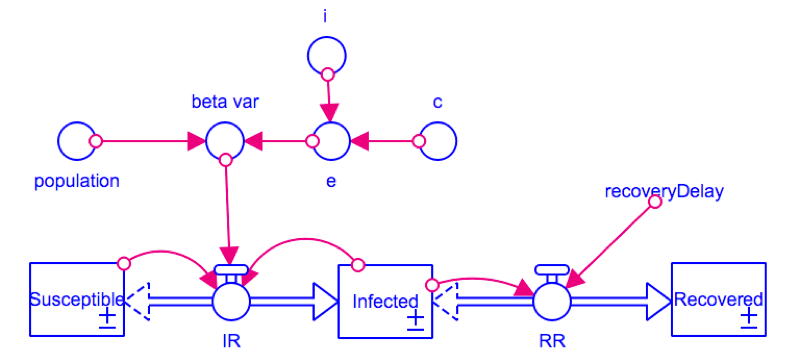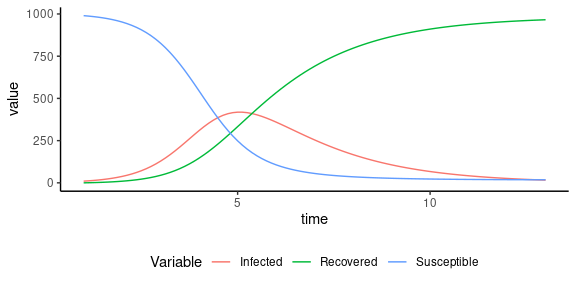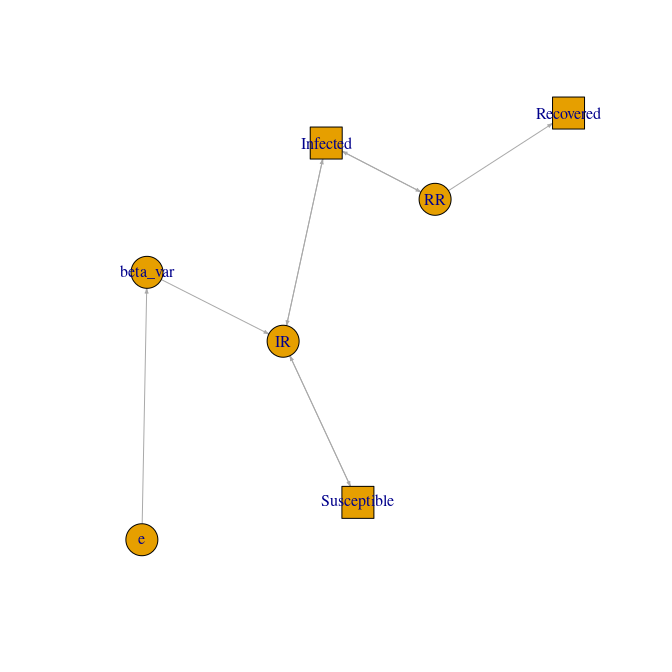## Model: SIR

The SIR model is an epidemiological model, due to Kermack and McKendrick, that computes the theoretical number of people infected with a contagious illness in a closed population over time. The SIR models the flows of people between three states:

Susceptible (S)
number of individuals who are not infected but could become infected.
Infected (I)
number of individuals who have the disease and can transmit it to the susceptibles.
Recovered (R)
number of individuals who have recovered from the disease and are immune from getting it again.

The model assumes a time scale short enough that births and deaths can be neglected.

The SIR model is used where individuals infect each other directly. Contact between people is also modeled to be random.

The rate that people become infected is proportional to the number of people who are infected, and the number of people who are susceptible. If there are lots of people infected, the chances of a susceptible coming into contact with someone who is infected is high. Likewise, if there are very few people who are susceptible, the chances of a susceptible coming into contact with an infected is lower (since most of the contact would be between either infected or recovered). In mathematical notation, the model is described by the following equations:

$\begin{equation} \frac{dS}{dt} = -\beta SI \end{equation}$

$\begin{equation} \frac{dI}{dt} = \beta SI - \frac{I}{rd} \end{equation}$

$\begin{equation} \frac{dR}{dt} = \frac{I}{rd} \end{equation}$

$\begin{equation} \beta = \frac{e}{n} \end{equation}$

$\begin{equation} e = c \times i \end{equation}$

$$n$$ denotes the population size; $$\beta$$ the per capita rate at which two specific individuals come into effective contact per unit time; $$e$$ the effective contacts per infected individual; $$c$$ the contacts per person per unit time; and $$i$$ the infectivity.

There exists several ways to implement this model. On one side of the spectrum, we find specialised software such as Vensim and Stella that offer friendly graphical user interfaces to seamlessly design complex models, emphasising on a systems perspective. Figure 1 presents the implementation of the SIR model in Stella.Figure 1. SIR

On the other side, we can implement the model in flexible and powerful statistical environments such as R that offer innumerable tools for numerical analysis and data visualisation. Specifically, models can be implemented with the deSolve library (see Duggan for more details). This alternative requires the user to type all model equations in computational order. For large models, this task can be cumbersome and may lead to incorrect implementations.

In order to bridge these two approaches, the package readsdr fills the gap by automating the translation from Vensim and Stella models to R. Such a process is achieved by the function read_xmile.

library(readsdr)

filepath <- system.file("models/", "SIR.stmx", package = "readsdr")
mdl      <- read_xmile(filepath) 

read_xmile returns a list of three elements. The element description contains the simulation parameters and the model variables as lists.

description <- mdl$description model_summary <- data.frame(n_stocks = length(description$levels),
n_variables = length(description$variables), n_consts = length(description$constants))
print(model_summary)
#>   n_stocks n_variables n_consts
#> 1        3           4        4

To simulate a model with deSolve, we use the function ode. This routine takes as arguments, a vector with the model’s stocks, the simulation time, the equations wrapped in a function, the model’s constants and the integration method. Indeed, the second element from read_xmile is a list with all these inputs but the integration method. If this is the only element of interest, readsdr provides xmile_to_deSolve. ode returns a matrix which then is converted to a data frame for convenience. readsdr offers sd_simulate to simplify this process.

deSolve_components <- mdl$deSolve_components all.equal(deSolve_components, xmile_to_deSolve(filepath)) #>  TRUE library(deSolve) simtime <- seq(deSolve_components$sim_params$start, deSolve_components$sim_params$stop, deSolve_components$sim_params$dt) output_deSolve <- ode(y = deSolve_components$stocks,
times  = simtime,
func   = deSolve_components$func, parms = deSolve_components$consts,
method = "euler")

result_df <- data.frame(output_deSolve)

#>    time Susceptible Infected Recovered        RR e beta_var       IR population
#> 1 1.000    990.0000 10.00000  0.000000  5.000000 2    0.002 19.80000       1000
#> 2 1.125    987.5250 11.85000  0.625000  5.925000 2    0.002 23.40434       1000
#> 3 1.250    984.5995 14.03492  1.365625  7.017459 2    0.002 27.63754       1000
#> 4 1.375    981.1448 16.61243  2.242807  8.306214 2    0.002 32.59839       1000
#> 5 1.500    977.0700 19.64895  3.281084  9.824476 2    0.002 38.39680       1000
#> 6 1.625    972.2704 23.22049  4.509144 11.610246 2    0.002 45.15319       1000
#>      i c recoveryDelay
#> 1 0.25 8             2
#> 2 0.25 8             2
#> 3 0.25 8             2
#> 4 0.25 8             2
#> 5 0.25 8             2
#> 6 0.25 8             2

result_df2 <- sd_simulate(deSolve_components)
identical(result_df, result_df2)
#>  TRUE

With the results in a data frame, we can manipulate the data structure for further analysis and data visualisation. In this case, we simply produce a plot with the behaviour over time using ggplot2, a library part of the tidyverse. See Duggan (2018) for more details.

library(dplyr)
library(tidyr)
library(ggplot2)

tidy_result_df <- result_df %>%
select(time, Susceptible, Infected, Recovered) %>%
pivot_longer(-time, names_to = "Variable")

ggplot(tidy_result_df, aes(x = time, y = value)) +
geom_line(aes(group = Variable, colour = Variable)) +
theme_classic() +
theme(legend.position = "bottom")Finally, the third element from read_xmile is a list of two data frames. These structures describe the model as a graph in terms of vertices and edges, and serve as input to the functions in igraph, a library collection for creating and manipulating graphs and analyzing networks.

library(igraph)

gr <- graph_from_data_frame(mdl$graph_dfs$edges, directed = TRUE,
vertices = mdl$graph_dfs$nodes)

V(gr)$shape <- ifelse(V(gr)$type == "stock", "rectangle", "circle")

plot.igraph(gr, edge.arrow.size = 0.25,
vertex.label.cex = 1, layout = layout.lgl(gr))